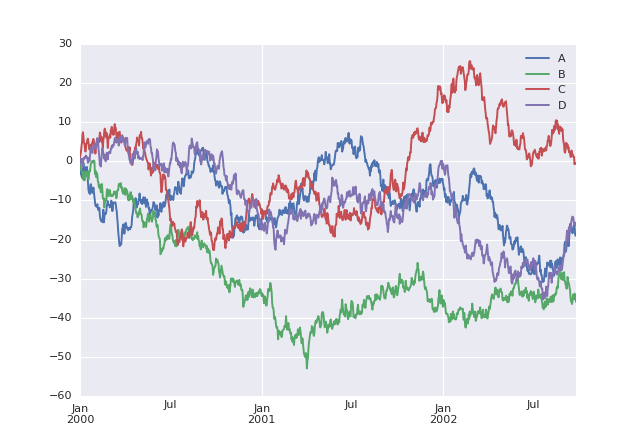%matplotlib notebook

import pandas as pd
import numpy as np
import matplotlib

from matplotlib import pyplot as plt
import seaborn as sns

ts = pd.Series(np.random.randn(1000), index=pd.date_range('1/1/2000', periods=1000))
ts = ts.cumsum()

df = pd.DataFrame(np.random.randn(1000, 4), index=ts.index,
columns=['A', 'B', 'C', 'D'])
df = df.cumsum()
df.plot(); plt.legend(loc='best')<matplotlib.legend.Legend at 0x7fbbc0978320>

% Steradian cone in sphere % Author: Bartman \documentclass[tikz,border=10pt]{standalone} %%%< \usepackage{verbatim} %%%> \begin{comment} :Title: Steradian cone in sphere :Tags: 3D;Angles;Intersections;Shadings;MMathematics;Geometry :Author: Bartman :Slug: steradian-cone-sphere

A graphical representation of a steradian. It is the solid angle subtended at the center of a unit sphere by a unit area on its surface. (Wikipedia)

The part of the cone is from http://tex.stackexchange.com/a/186109/213 \end{comment} \usepackage{sansmath} \usetikzlibrary{shadings,intersections} \begin{document} \begin{tikzpicture}[font = \sansmath] \coordinate (O) at (0,0);

% ball background color \shade[ball color = blue, opacity = 0.2] (0,0) circle [radius = 2cm];

% cone \begin{scope} \def\rx{0.71}% horizontal radius of the ellipse \def\ry{0.15}% vertical radius of the ellipse \def\z{0.725}% distance from center of ellipse to origin

\path [name path = ellipse]    (0,\z) ellipse ({\rx} and {\ry});
\path [name path = horizontal] (-\rx,\z-\ry*\ry/\z)
-- (\rx,\z-\ry*\ry/\z);
\path [name intersections = {of = ellipse and horizontal}];

% radius to base of cone in ball
\draw[fill = gray!50, gray!50] (intersection-1) -- (0,0)
-- (intersection-2) -- cycle;
% base of cone in ball
\draw[fill = gray!30, densely dashed] (0,\z) ellipse ({\rx} and {\ry});


\end{scope}

% label of cone \draw (0.25,0.4) -- (0.9,0.1) node at (1.05,0.0) {$q$};

% ball \draw (O) circle [radius=2cm]; % label of ball center point \filldraw (O) circle (1pt) node[below] {$P$};

% radius \draw[densely dashed] (O) to [edge label = $r$] (-1.33,1.33); \draw[densely dashed] (O) -- (1.33,1.33);

% cut of ball surface \draw[red] (-1.35,1.47) arc [start angle = 140, end angle = 40, x radius = 17.6mm, y radius = 14.75mm]; \draw[red, densely dashed] (-1.36,1.46) arc [start angle = 170, end angle = 10, x radius = 13.8mm, y radius = 3.6mm]; \draw[red] (-1.29,1.52) arc [start angle=-200, end angle = 20, x radius = 13.75mm, y radius = 3.15mm];

% label of cut of ball surface \draw (-1.2,2.2) -- (-0.53,1.83) node at (-1.37,2.37) {$A$}; \end{tikzpicture} \end{document}

% Steradian cone in sphere % Author: Bartman \documentclass[tikz,border=10pt]{standalone} %%%< \usepackage{verbatim} %%%> \begin{comment} :Title: Steradian cone in sphere :Tags: 3D;Angles;Intersections;Shadings;MMathematics;Geometry :Author: Bartman :Slug: steradian-cone-sphere A graphical representation of a steradian. It is the solid angle subtended at the center of a unit sphere by a unit area on its surface. (Wikipedia) Made by Bartman on http://golatex.de/3d-kugel-in-tikz-t17380.html The part of the cone is from http://tex.stackexchange.com/a/186109/213 \end{comment} \usepackage{sansmath} \usetikzlibrary{shadings,intersections} \begin{document} \begin{tikzpicture}[font = \sansmath] \coordinate (O) at (0,0); % ball background color \shade[ball color = blue, opacity = 0.2] (0,0) circle [radius = 2cm]; % cone \begin{scope} \def\rx{0.71}% horizontal radius of the ellipse \def\ry{0.15}% vertical radius of the ellipse \def\z{0.725}% distance from center of ellipse to origin \path [name path = ellipse] (0,\z) ellipse ({\rx} and {\ry}); \path [name path = horizontal] (-\rx,\z-\ry*\ry/\z) -- (\rx,\z-\ry*\ry/\z); \path [name intersections = {of = ellipse and horizontal}]; % radius to base of cone in ball \draw[fill = gray!50, gray!50] (intersection-1) -- (0,0) -- (intersection-2) -- cycle; % base of cone in ball \draw[fill = gray!30, densely dashed] (0,\z) ellipse ({\rx} and {\ry}); \end{scope} % label of cone \draw (0.25,0.4) -- (0.9,0.1) node at (1.05,0.0) {$q$}; % ball \draw (O) circle [radius=2cm]; % label of ball center point \filldraw (O) circle (1pt) node[below] {$P$}; % radius \draw[densely dashed] (O) to [edge label = $r$] (-1.33,1.33); \draw[densely dashed] (O) -- (1.33,1.33); % cut of ball surface \draw[red] (-1.35,1.47) arc [start angle = 140, end angle = 40, x radius = 17.6mm, y radius = 14.75mm]; \draw[red, densely dashed] (-1.36,1.46) arc [start angle = 170, end angle = 10, x radius = 13.8mm, y radius = 3.6mm]; \draw[red] (-1.29,1.52) arc [start angle=-200, end angle = 20, x radius = 13.75mm, y radius = 3.15mm]; % label of cut of ball surface \draw (-1.2,2.2) -- (-0.53,1.83) node at (-1.37,2.37) {$A$}; \end{tikzpicture} \end{document}